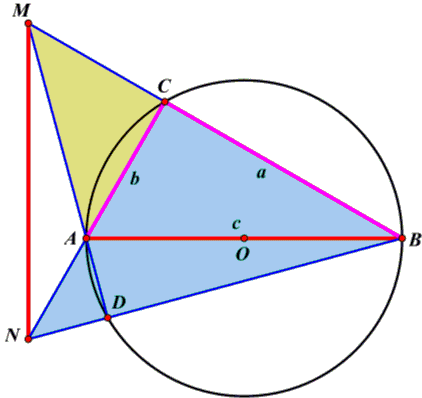# A Proof of the Pythagorean Theorem with Orthocenter and Right Isosceles Triangles

### Quang Tuan Bui

ABC is a triangle right at C with sides a, b, c and circumcircle (O) centered at O, the midpoint of the hypotenuse AB. Suppose b ≤ a.

Construct point M on the ray BC such that CM = AC = b and M is outside segment BC. The line AM intersects circle (O) again at D. Since b ≤ a D is on the semicircle other than the semicircle which contains C.The intersection N of AC and BD is on the ray BD and is outside the segment BD. Therefore:

Area(ΔBMN) = Area(ΔMNA) + Area(ΔCNB) + Area(ΔCMA)
Area(ΔBMN) - Area(ΔMNA) = Area(ΔCNB) + Area(ΔCMA)

It follows that A is the orthocenter of ΔBMN, implying

 (1) AB·MN/2 = Area(ΔCNB) + Area(ΔCMA).

ΔMCA is right isosceles suh that triangles NDA, NCB, MDB are also right isosceles.

Two right triangles CMN and CAB are congruent (because CM = CA, CN = CB) therefore MN = AB = c and from (1) we have:

c²/2 = Area(ΔCNB) + Area(ΔCMA)
c²/2 = a²/2 + b²/2
c² = a² + b².

which is the Pythagorean identity.### The Orthocenter